Tamil Nadu Board of Secondary EducationSSLC (English Medium) Class 6th

# Tamil Nadu Board Samacheer Kalvi solutions for Class 6th Mathematics Term 2 Answers Guide chapter 4 - Geometry [Latest edition]

#### Chapters## Chapter 4: Geometry

Exercise 4.1Exercise 4.2Exercise 4.3
Exercise 4.1 [Pages 65 - 67]

### Tamil Nadu Board Samacheer Kalvi solutions for Class 6th Mathematics Term 2 Answers Guide Chapter 4 GeometryExercise 4.1 [Pages 65 - 67]

#### Fill in the blanks:

Exercise 4.1 | Q 1. a) | Page 65

Every triangle has at least ________ acute angles

Exercise 4.1 | Q 1. b) | Page 65

A triangle in which none of the sides equal is called a _________

Exercise 4.1 | Q 1. c) | Page 65

In an isosceles triangle ________ angles are equal

Exercise 4.1 | Q 1. d) | Page 65

The sum of three angles of a triangle is __________

Exercise 4.1 | Q 1. e) | Page 65

A right angled triangle with two equal sides is called ________

Exercise 4.1 | Q 2 | Page 65

Match the following:

 Column A Column B (i) No sides are equal Isosceles triangle (ii) One right angle Scalene triangle (iii) One obtuse angle Right angled triangle (iv) Two sides of equal length Equilateral triangle (v) All sides are equal Obtuse angled triangle
Exercise 4.1 | Q 3 | Page 65

In ΔABC, name the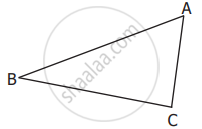a) Three sides: _________, __________, __________
b) Three Angles: _________, __________, __________
c) Three Vertices: _________, __________, __________

Exercise 4.1 | Q 4. i) | Page 66

Classify the given triangle based on its sides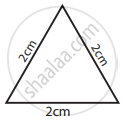• Scalene triangle

• Isosceles triangle

• Equilateral triangle

Exercise 4.1 | Q 4. ii) | Page 66

Classify the given triangle based on its sides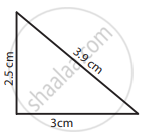• Scalene triangle

• Isosceles triangle

• Equilateral triangle

Exercise 4.1 | Q 4. iii) | Page 66

Classify the given triangle based on its sides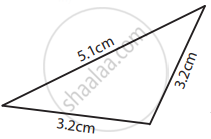• Scalene triangle

• Isosceles triangle

• Equilateral triangle

Exercise 4.1 | Q 4. iv) | Page 66

Classify the given triangle based on its sides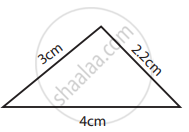• Scalene triangle

• Isosceles triangle

• Equilateral triangle

Exercise 4.1 | Q 5. i) | Page 66

Classify the given triangle based on its angles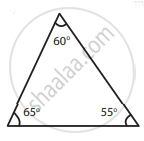• Acute angled triangle

• Right angled triangle

• Obtuse angled triangle

Exercise 4.1 | Q 5. ii) | Page 66

Classify the given triangle based on its angles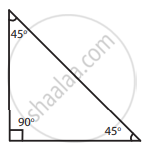• Acute angled triangle

• Right angled triangle

• Obtuse angled triangle

Exercise 4.1 | Q 5. iii) | Page 66

Classify the given triangle based on its angles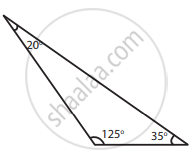• Acute angled triangle

• Right angled triangle

• Obtuse angled triangle

Exercise 4.1 | Q 5. iv) | Page 66

Classify the given triangle based on its angles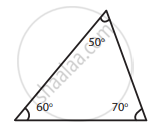• Acute angled triangle

• Right angled triangle

• Obtuse angled triangle

Exercise 4.1 | Q 6. (i) | Page 66

Classify the following triangle based on its sides and angles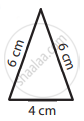Exercise 4.1 | Q 6. (ii) | Page 66

Classify the following triangle based on its sides and angles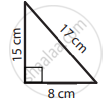Exercise 4.1 | Q 6. (iii) | Page 66

Classify the following triangle based on its sides and angles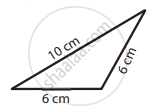Exercise 4.1 | Q 6. (iv) | Page 66

Classify the following triangle based on its sides and angles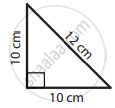Exercise 4.1 | Q 6. (v) | Page 66

Classify the following triangle based on its sides and angles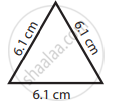Exercise 4.1 | Q 6. (vi) | Page 66

Classify the following triangle based on its sides and angles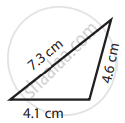Exercise 4.1 | Q 7. (i) | Page 66

Can a triangle be formed with the following sides? If yes, name the type of triangle.

8 cm, 6 cm, 4 cm

Exercise 4.1 | Q 7. (ii) | Page 66

Can a triangle be formed with the following sides? If yes, name the type of triangle.

10 cm, 8 cm, 5 cm

Exercise 4.1 | Q 7. (iii) | Page 66

Can a triangle be formed with the following sides? If yes, name the type of triangle.

6.2 cm, 1.3 cm, 3.5 cm

Exercise 4.1 | Q 7. (iv) | Page 66

Can a triangle be formed with the following sides? If yes, name the type of triangle.

6 cm, 6 cm, 4 cm

Exercise 4.1 | Q 7. (v) | Page 66

Can a triangle be formed with the following sides? If yes, name the type of triangle.

3.5 cm, 3.5 cm, 3.5 cm

Exercise 4.1 | Q 7. (vi) | Page 66

Can a triangle be formed with the following sides? If yes, name the type of triangle.

9 cm, 4 cm, 5 cm

Exercise 4.1 | Q 8. (i) | Page 66

Can a triangle be formed with the following angles? If yes, name the type of triangle.

60°, 60°, 60°

Exercise 4.1 | Q 8. (ii) | Page 66

Can a triangle be formed with the following angles? If yes, name the type of triangle.

90°, 55°, 35°

Exercise 4.1 | Q 8. (iii) | Page 66

Can a triangle be formed with the following angles? If yes, name the type of triangle.

60°, 40°, 42°

Exercise 4.1 | Q 8. (iv) | Page 66

Can a triangle be formed with the following angles? If yes, name the type of triangle.

60°, 90°, 90°

Exercise 4.1 | Q 8. (v) | Page 66

Can a triangle be formed with the following angles? If yes, name the type of triangle.

70°, 60°, 50°

Exercise 4.1 | Q 8. (vi) | Page 66

Can a triangle be formed with the following angles? If yes, name the type of triangle.

100°, 50°, 30°

Exercise 4.1 | Q 9. (i) | Page 66

Two angles of the triangle are given. Find the third angle.

80°, 60°

Exercise 4.1 | Q 9. (ii) | Page 66

Two angles of the triangle are given. Find the third angle.

75°, 35°

Exercise 4.1 | Q 9. (iii) | Page 66

Two angles of the triangle are given. Find the third angle.

52°, 68°

Exercise 4.1 | Q 9. (iv) | Page 66

Two angles of the triangle are given. Find the third angle.

50°, 90°

Exercise 4.1 | Q 9. (v) | Page 66

Two angles of the triangle are given. Find the third angle.

120°, 30°

Exercise 4.1 | Q 9. (vi) | Page 66

Two angles of the triangle are given. Find the third angle.

55°, 85°

Exercise 4.1 | Q 10 | Page 66

I am a closed figure with each of my three angles is 60°. Who am I?

Exercise 4.1 | Q 11 | Page 67

Using the given information, write the type of triangle in the table given below

 S.No ∠1 ∠2 ∠3 Type of triangle based on angles Type of triangle based on sides i. 60° 40° 80° Acute angled triangle. Scalene Triangle ii. 50° 50° 80° iii. 45° 45° 90° iv. 55° 45° 80° v. 75° 35° 70° vi. 60° 30° 90° vii. 25° 64° 91° viii. 120° 30° 30°

#### Objective Type Questions

Exercise 4.1 | Q 12 | Page 67

The given triangle is _________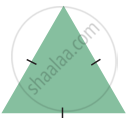• a right angled triangle

• an equilateral triangle

• a scalene triangle

• an obtuse angled triangle

Exercise 4.1 | Q 13 | Page 67

If all angles of a triangle are less than a right angle, then it is called ________

• an obtuse angled triangle

• a right angled triangle

• an isosceles right angled triangle

• an acute angled triangle

Exercise 4.1 | Q 14 | Page 67

If two sides of a triangle are 5 cm and 9 cm, then the third side is ________

• 5 cm

• 3 cm

• 4 cm

• 14 cm

Exercise 4.1 | Q 15 | Page 67

The angles of a right angled triangle are

• acute, acute, obtuse

• acute, right, right

• right, obtuse, acute

• acute, acute, right

Exercise 4.1 | Q 16 | Page 67

An equilateral triangle is

• an obtuse angled triangle

• a right angled triangle

• an acute angled triangle

• a scalene triangle

Exercise 4.2 [Page 73]

### Tamil Nadu Board Samacheer Kalvi solutions for Class 6th Mathematics Term 2 Answers Guide Chapter 4 GeometryExercise 4.2 [Page 73]

Exercise 4.2 | Q 1 | Page 73

Draw a line segment AB = 7 cm and mark a point P on it. Draw a line perpendicular to the given line segment at P

Exercise 4.2 | Q 2 | Page 73

Draw a line segment LM = 6.5 cm and take a point P not lying on it. Using a set square construct a line perpendicular to LM through P

Exercise 4.2 | Q 3 | Page 73

Find the distance between the given lines using a set square at two different points on each of the pairs of lines and check whether they are parallel.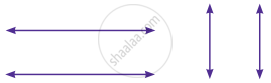Exercise 4.2 | Q 4 | Page 73

Draw a line segment measuring 7.8 cm. Mark a point B above it at a distance of 5 cm. Through B draw a line parallel to the given line segment

Exercise 4.2 | Q 5 | Page 73

Draw a line and mark a point R below it at a distance of 5.4 cm Through R draw a line parallel to the given line

Exercise 4.3 [Pages 73 - 74]

### Tamil Nadu Board Samacheer Kalvi solutions for Class 6th Mathematics Term 2 Answers Guide Chapter 4 GeometryExercise 4.3 [Pages 73 - 74]

#### Miscellaneous Practice Problems

Exercise 4.3 | Q 1 | Page 73

What are the angles of an isosceles right angled triangle?

Exercise 4.3 | Q 2 | Page 73

Which of the following correctly describes the given triangle?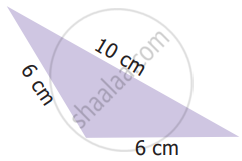• It is a right isosceles triangle

• It is an acute isosceles triangle

• It is an obtuse isosceles triangle

• It is an obtuse scalene triangle.

Exercise 4.3 | Q 3 | Page 73

Which of the following is not possible?

• An obtuse isosceles triangle

• An acute isosceles triangle

• An obtuse equilateral triangle

• An acute equilateral triangle

Exercise 4.3 | Q 4 | Page 73

If one angle of an isosceles triangle is 124°, then find the other angles

Exercise 4.3 | Q 5 | Page 73

The diagram shows a square ABCD. If the line segment joins A and C, then mention the type of triangles so formed.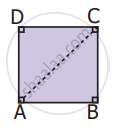Exercise 4.3 | Q 6 | Page 73

Draw a line segment AB of length 6 cm. At each end of this line segment AB, draw a line perpendicular to the line AB. Are these lines parallel?

#### Challenge Problems

Exercise 4.3 | Q 7 | Page 73

Is a triangle possible with the angles 90°, 90° and 0°? Why?

Exercise 4.3 | Q 8. (a) | Page 73

State the following statement is true or false? Why?

Every equilateral triangle is an isosceles triangle

• True

• False

Exercise 4.3 | Q 8. (b) | Page 73

State the following statement is true or false? Why?

Every isosceles triangle is an equilateral triangle

• True

• False

Exercise 4.3 | Q 9 | Page 74

If one angle of an isosceles triangle is 70°, then find the possibilities for the other two angles

Exercise 4.3 | Q 10 | Page 74

Which of the following can be the sides of an isosceles triangle?

• 6 cm, 3 cm, 3 cm

• 5 cm, 2 cm, 2 cm

• 6 cm, 6 cm, 7 cm

• 4 cm, 4 cm, 8 cm

Exercise 4.3 | Q 11 | Page 74

Study the given figure and identify the following triangles.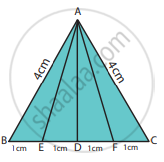(a) equilateral triangle
(b) isosceles triangles
(c) scalene triangles
(d) acute triangles
(e) obtuse triangles
(f) right triangles

Exercise 4.3 | Q 12 | Page 74

Two sides of the triangle are given in the table. Find the third side of the triangle.

 SI. No. Side - 1 Side - 2 The length of the third side (any three measures) i. 7 cm 4 cm ii. 8 cm 8 cm iii. 7.5 cm 3.5 cm iv. 10 cm 14 cm
Exercise 4.3 | Q 13 | Page 74

Complete the following table:

 Types of Triangle/Its Angles Acute angled triangle Right angled triangle Obtuse angled triangle Any two angles Always acute angles i. Always acute angles Third angle ii. Right angle iii.

## Chapter 4: Geometry

Exercise 4.1Exercise 4.2Exercise 4.3## Tamil Nadu Board Samacheer Kalvi solutions for Class 6th Mathematics Term 2 Answers Guide chapter 4 - Geometry

Tamil Nadu Board Samacheer Kalvi solutions for Class 6th Mathematics Term 2 Answers Guide chapter 4 (Geometry) include all questions with solution and detail explanation. This will clear students doubts about any question and improve application skills while preparing for board exams. The detailed, step-by-step solutions will help you understand the concepts better and clear your confusions, if any. Shaalaa.com has the Tamil Nadu Board of Secondary Education Class 6th Mathematics Term 2 Answers Guide solutions in a manner that help students grasp basic concepts better and faster.

Further, we at Shaalaa.com provide such solutions so that students can prepare for written exams. Tamil Nadu Board Samacheer Kalvi textbook solutions can be a core help for self-study and acts as a perfect self-help guidance for students.

Concepts covered in Class 6th Mathematics Term 2 Answers Guide chapter 4 Geometry are Concept of Triangles - Sides, Angles, Vertices, Interior and Exterior of Triangle, Classification of Triangles (On the Basis of Sides, and of Angles), Equilateral Triangle, Isosceles Triangles, Scalene Triangle, Acute Angled Triangle, Obtuse Angled Triangle, Triangle Inequality, Right Angled Triangle, Properties of a Triangle, Drawing a Perpendicular to a Line at a Point on the Line, Drawing a Perpendicular to a Line Through a Point Not on It, Construction of Parallel Lines.

Using Tamil Nadu Board Samacheer Kalvi Class 6th solutions Geometry exercise by students are an easy way to prepare for the exams, as they involve solutions arranged chapter-wise also page wise. The questions involved in Tamil Nadu Board Samacheer Kalvi Solutions are important questions that can be asked in the final exam. Maximum students of Tamil Nadu Board of Secondary Education Class 6th prefer Tamil Nadu Board Samacheer Kalvi Textbook Solutions to score more in exam.

Get the free view of chapter 4 Geometry Class 6th extra questions for Class 6th Mathematics Term 2 Answers Guide and can use Shaalaa.com to keep it handy for your exam preparation